# Describing Motion and Forces Newtons Laws Motion Motion

• Slides: 29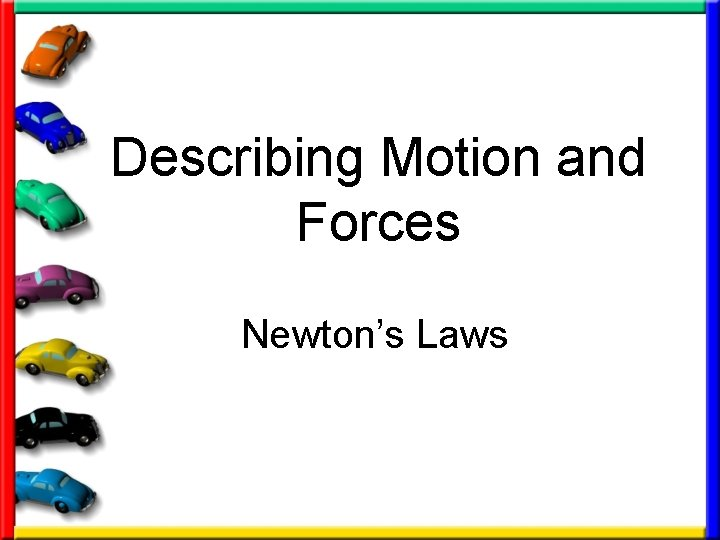Describing Motion and Forces Newton’s LawsMotion • Motion – an object’s change in position relative to a reference pointReference Point ►The Earth’s surface is used as a common reference point ►A moving object can be used as a reference point as wellMeasuring Distance • Meter – international unit for measuring distance. 1 mm = 50 m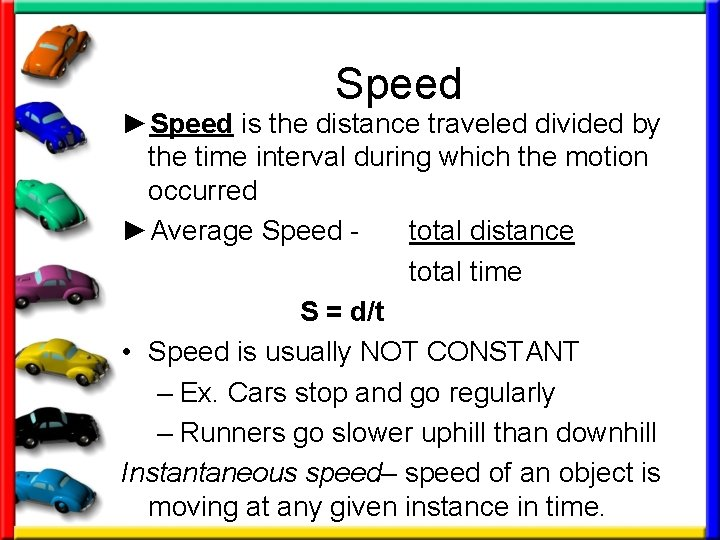Speed ►Speed is the distance traveled divided by the time interval during which the motion occurred ►Average Speed total distance total time S = d/t • Speed is usually NOT CONSTANT – Ex. Cars stop and go regularly – Runners go slower uphill than downhill Instantaneous speed– speed of an object is moving at any given instance in time.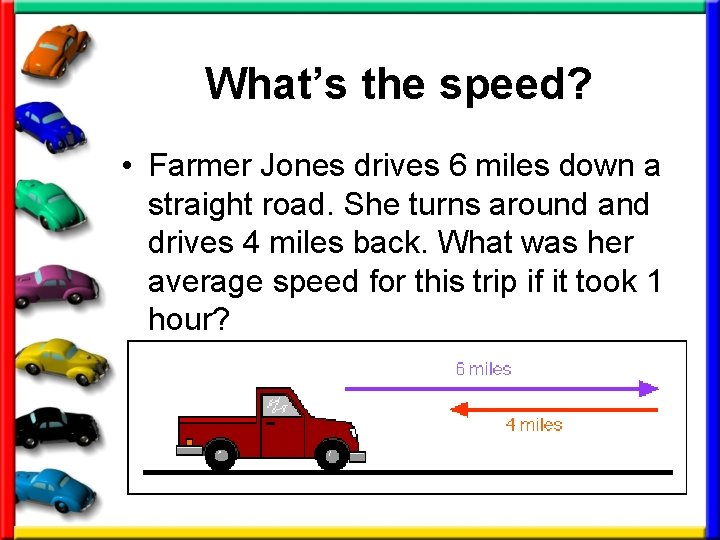What’s the speed? • Farmer Jones drives 6 miles down a straight road. She turns around and drives 4 miles back. What was her average speed for this trip if it took 1 hour?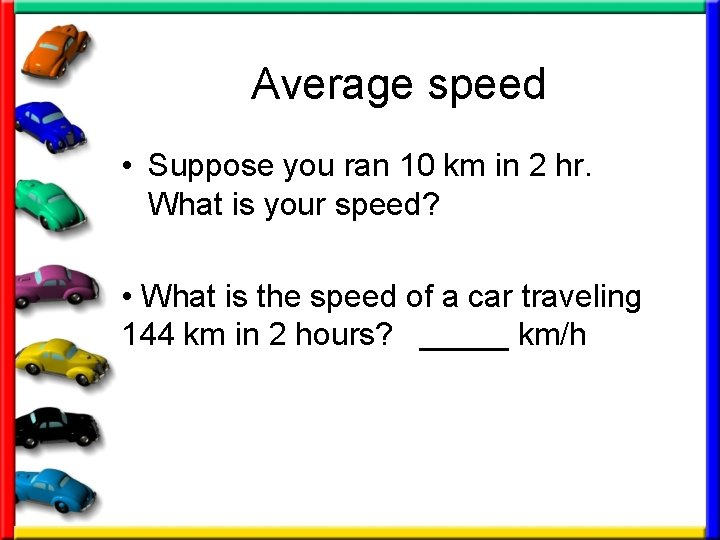Average speed • Suppose you ran 10 km in 2 hr. What is your speed? • What is the speed of a car traveling 144 km in 2 hours? _____ km/h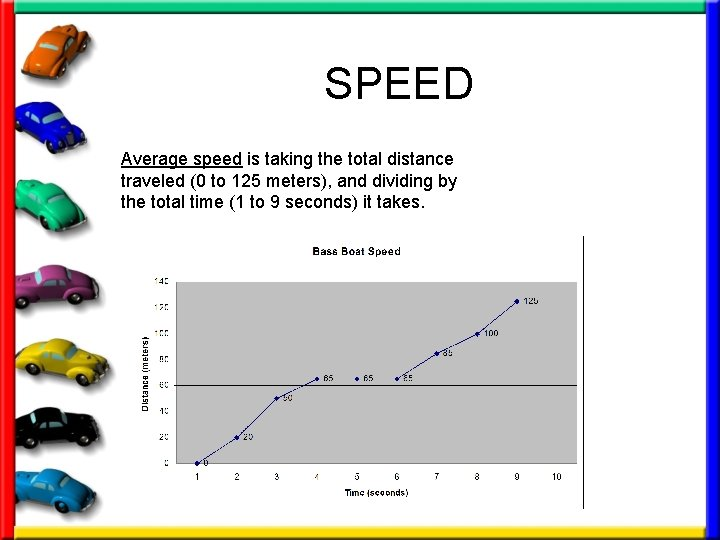SPEED Average speed is taking the total distance traveled (0 to 125 meters), and dividing by the total time (1 to 9 seconds) it takes.Velocity VELOCITY– speed in a given direction Imagine two birds leave the same tree at the same time. The both fly at 10 km/hr for 5 min. Why don’t they end up at the same place? • Indicate which of the following are velocities: a. 125 cm/sec b. 30 km/h northwest c. 350 m/sec north d. 520 km/h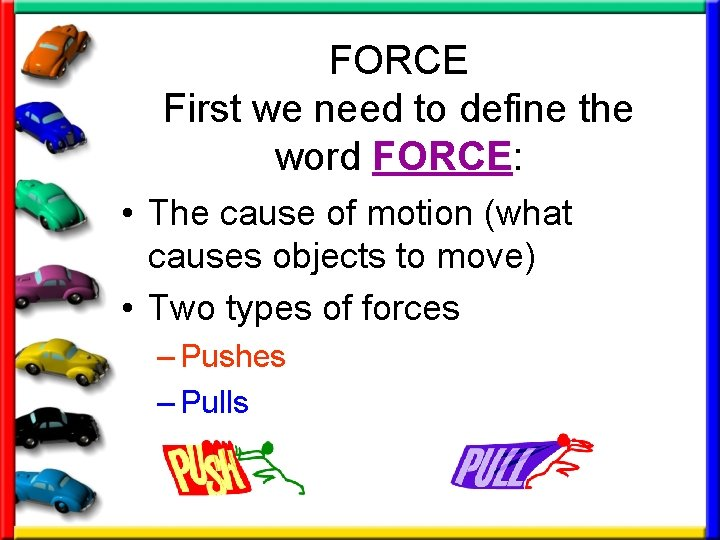FORCE First we need to define the word FORCE: • The cause of motion (what causes objects to move) • Two types of forces – Pushes – Pulls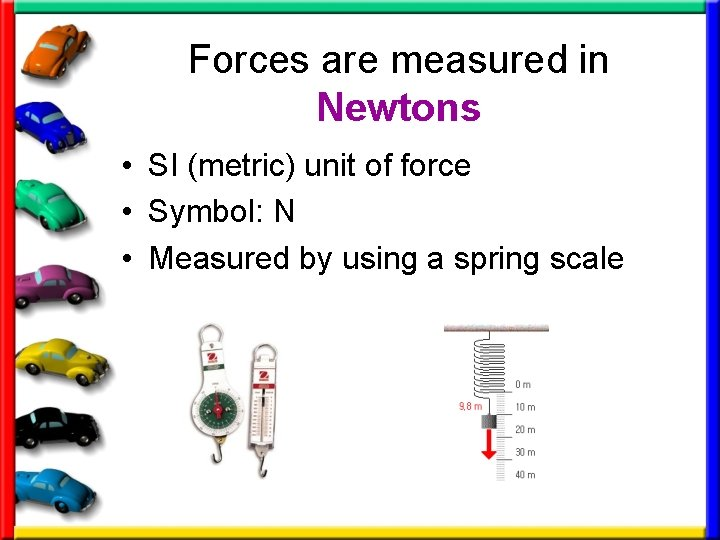Forces are measured in Newtons • SI (metric) unit of force • Symbol: N • Measured by using a spring scale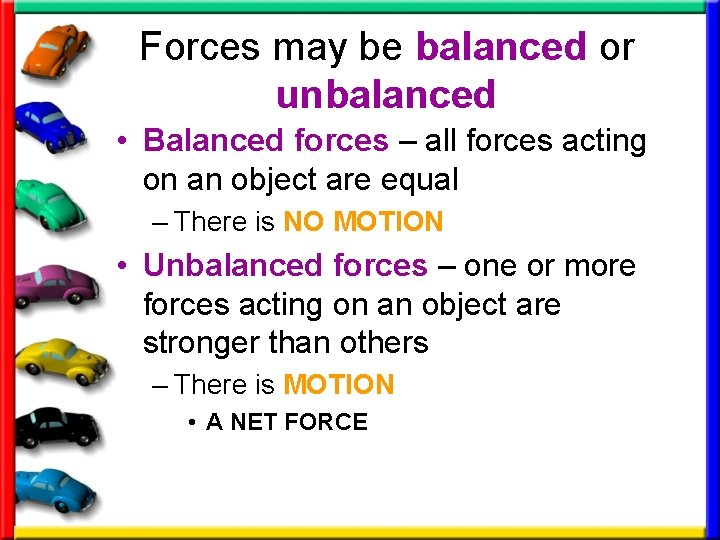Forces may be balanced or unbalanced • Balanced forces – all forces acting on an object are equal – There is NO MOTION • Unbalanced forces – one or more forces acting on an object are stronger than others – There is MOTION • A NET FORCE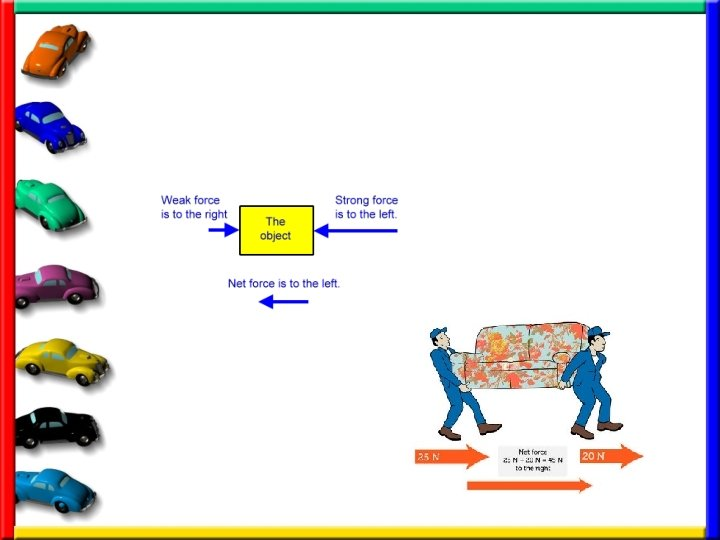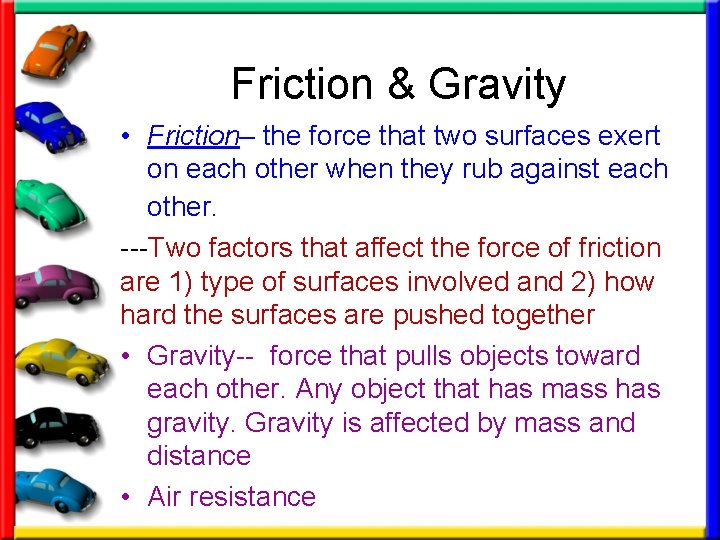Friction & Gravity • Friction– the force that two surfaces exert on each other when they rub against each other. ---Two factors that affect the force of friction are 1) type of surfaces involved and 2) how hard the surfaces are pushed together • Gravity-- force that pulls objects toward each other. Any object that has mass has gravity. Gravity is affected by mass and distance • Air resistance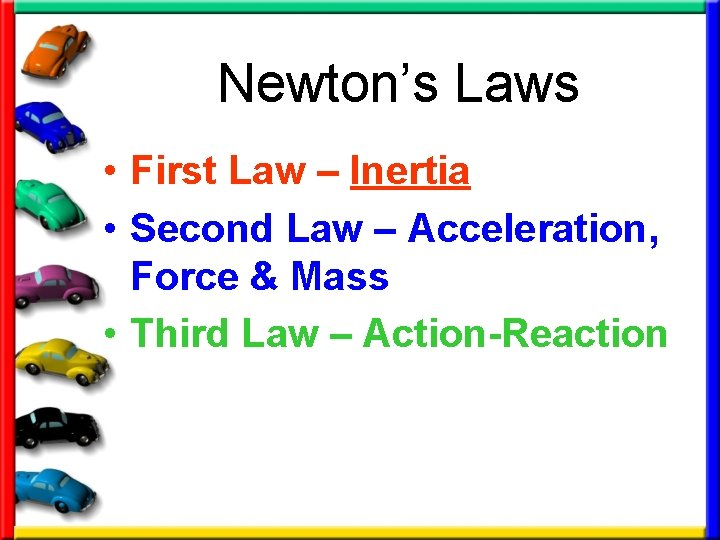Newton’s Laws • First Law – Inertia • Second Law – Acceleration, Force & Mass • Third Law – Action-Reaction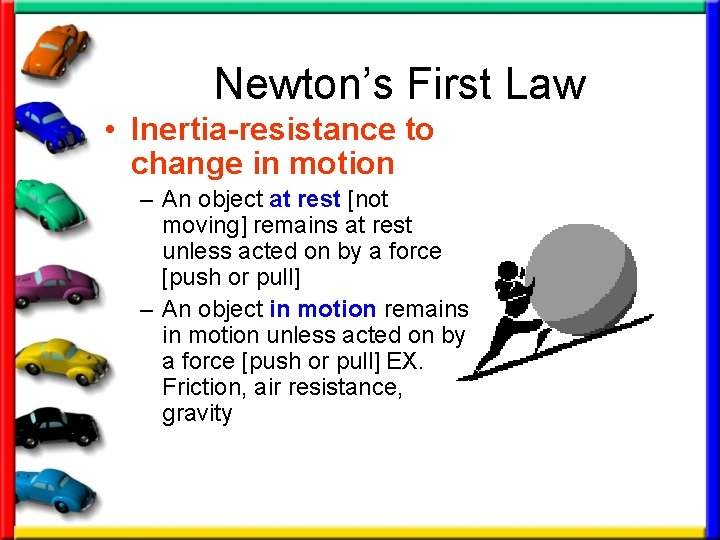Newton’s First Law • Inertia-resistance to change in motion – An object at rest [not moving] remains at rest unless acted on by a force [push or pull] – An object in motion remains in motion unless acted on by a force [push or pull] EX. Friction, air resistance, gravity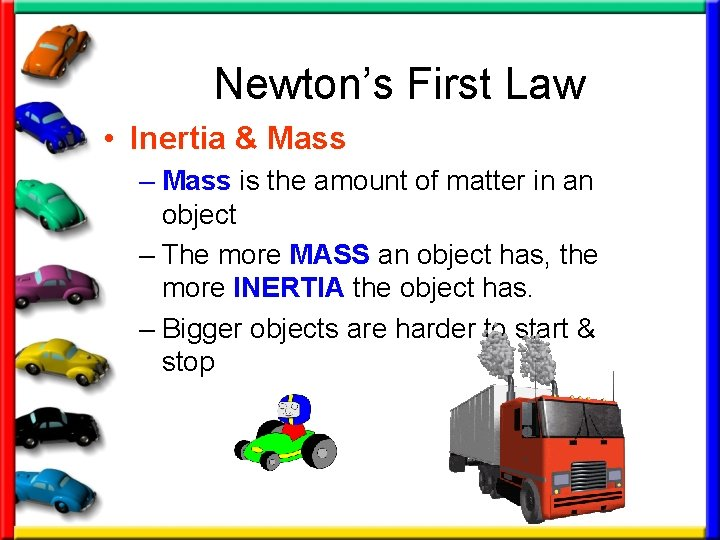Newton’s First Law • Inertia & Mass – Mass is the amount of matter in an object – The more MASS an object has, the more INERTIA the object has. – Bigger objects are harder to start & stopNewton’s 1 st Law • http: //teachertech. rice. edu/Participan ts/louviere/Newton/law 1. html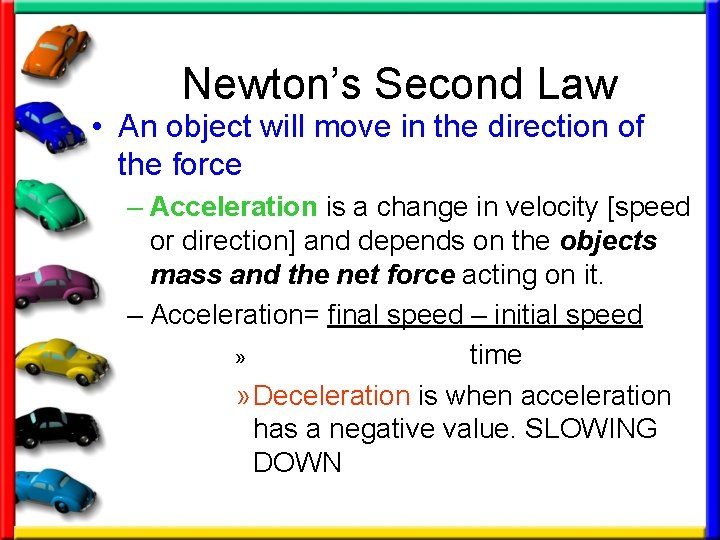Newton’s Second Law • An object will move in the direction of the force – Acceleration is a change in velocity [speed or direction] and depends on the objects mass and the net force acting on it. – Acceleration= final speed – initial speed » time » Deceleration is when acceleration has a negative value. SLOWING DOWN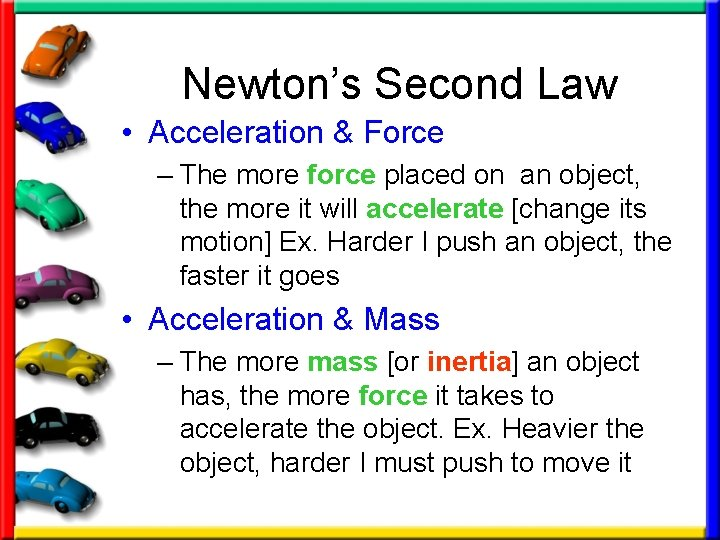Newton’s Second Law • Acceleration & Force – The more force placed on an object, the more it will accelerate [change its motion] Ex. Harder I push an object, the faster it goes • Acceleration & Mass – The more mass [or inertia] an object has, the more force it takes to accelerate the object. Ex. Heavier the object, harder I must push to move it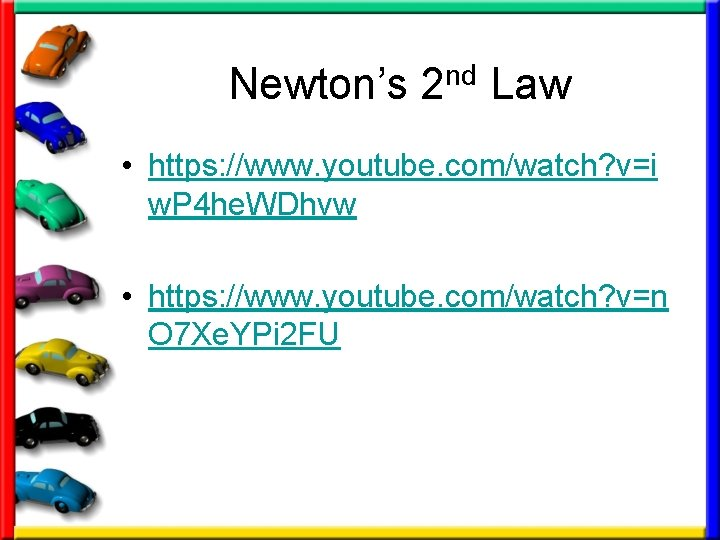Newton’s 2 nd Law • https: //www. youtube. com/watch? v=i w. P 4 he. WDhvw • https: //www. youtube. com/watch? v=n O 7 Xe. YPi 2 FU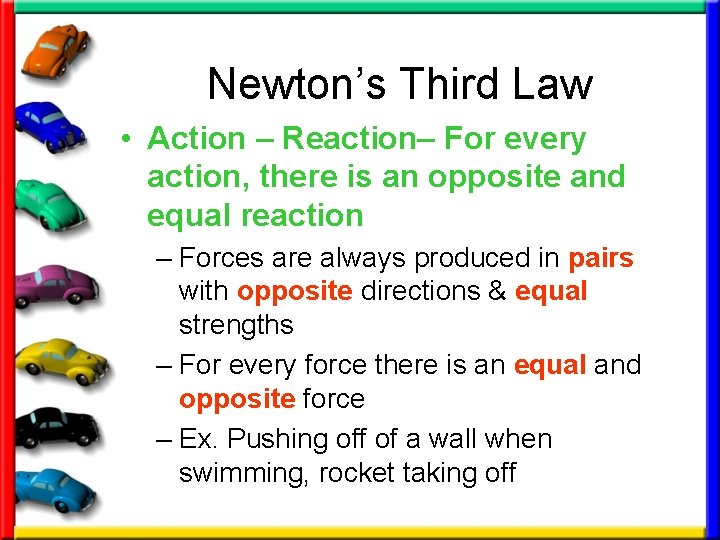Newton’s Third Law • Action – Reaction– For every action, there is an opposite and equal reaction – Forces are always produced in pairs with opposite directions & equal strengths – For every force there is an equal and opposite force – Ex. Pushing off of a wall when swimming, rocket taking offNewton’s 3 rd Law • https: //www. youtube. com/watch? v=Q q. Kg. B 1 o. Hrm. A • https: //www. youtube. com/watch? v=8 b. Td. Mm. NZm 2 M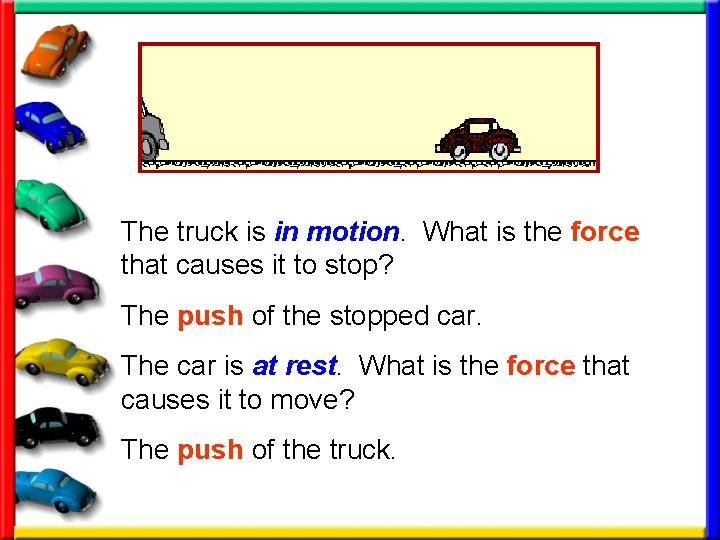The truck is in motion. What is the force that causes it to stop? The push of the stopped car. The car is at rest. What is the force that causes it to move? The push of the truck.What about the ladder on top of the truck? The ladder is in motion because the truck is in motion. When the truck stops, the ladder stays in motion. The truck is stopped by the force of the car, but the ladder is not. What force stops the ladder? Gravity.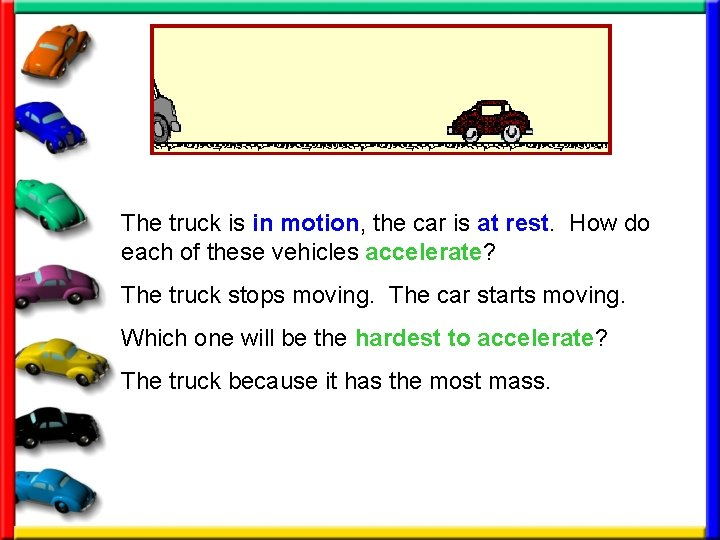The truck is in motion, the car is at rest. How do each of these vehicles accelerate? The truck stops moving. The car starts moving. Which one will be the hardest to accelerate? The truck because it has the most mass.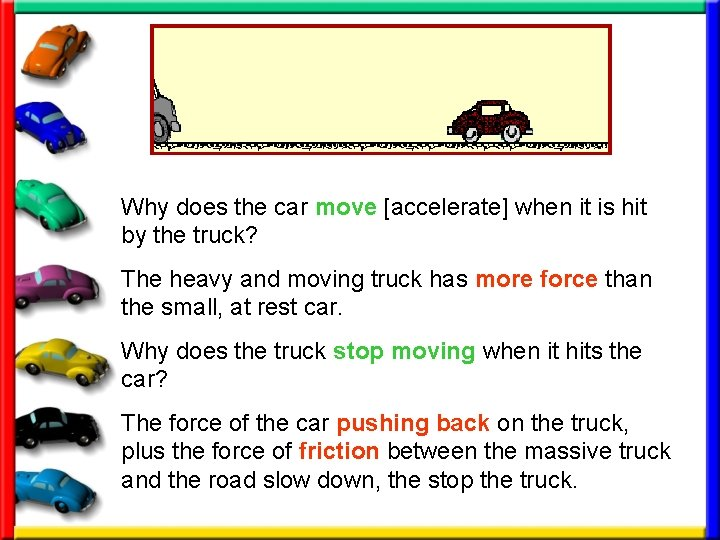Why does the car move [accelerate] when it is hit by the truck? The heavy and moving truck has more force than the small, at rest car. Why does the truck stop moving when it hits the car? The force of the car pushing back on the truck, plus the force of friction between the massive truck and the road slow down, the stop the truck.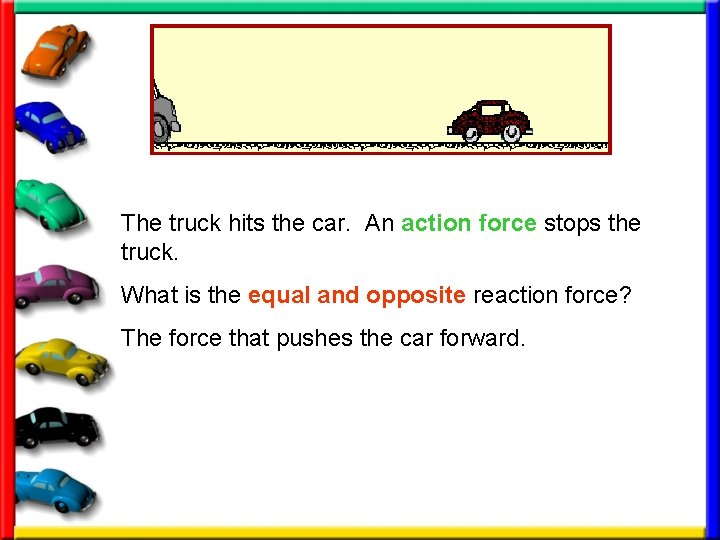The truck hits the car. An action force stops the truck. What is the equal and opposite reaction force? The force that pushes the car forward.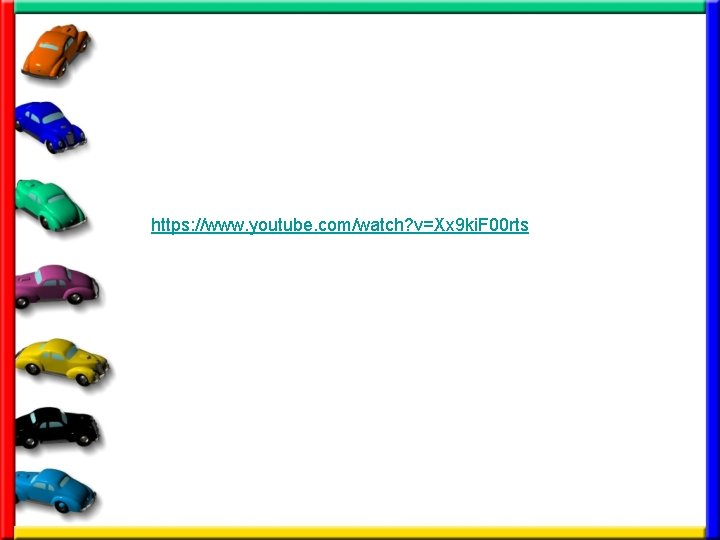https: //www. youtube. com/watch? v=Xx 9 ki. F 00 rts